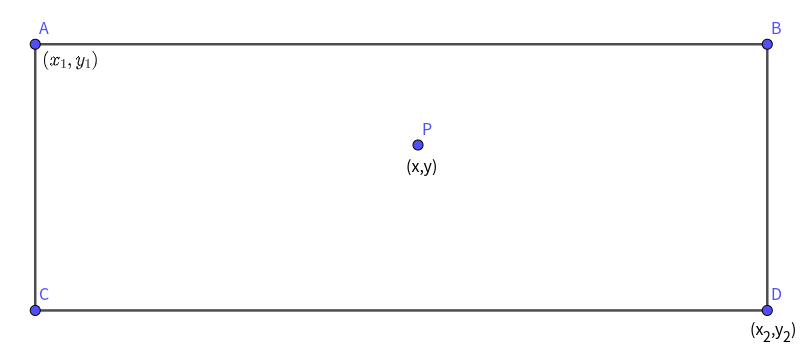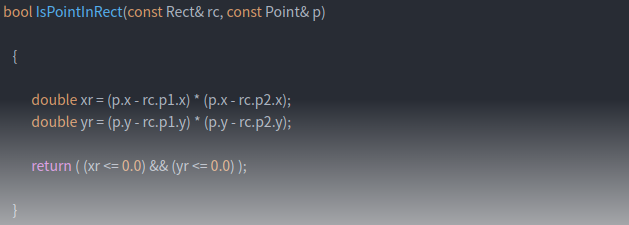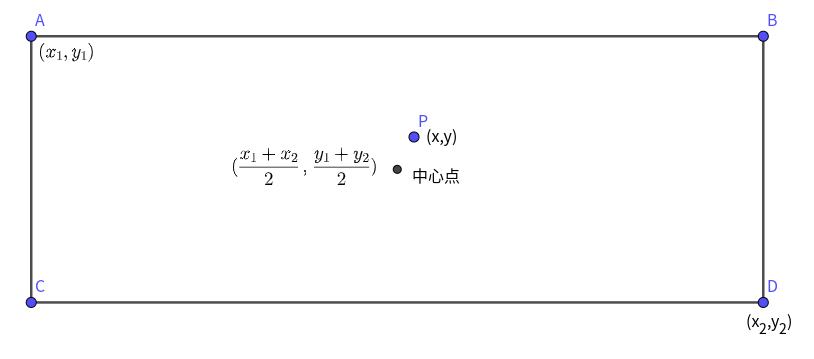• 如何判断点在矩形内
2022-08-13 17:29:17

1、判断点是否在矩形内部

bool pointInRect(Point2f pt, Rect rect)
{
if ((pt.x > rect.x) && (pt.y > rect.y) && (pt.x < (rect.x + rect.width)) && (pt.y < (rect.y + rect.height)))
{
return true;
}
return false;
}

2、判断两个矩形是否相交，并且判断一个矩形是否在另一个矩形内部

bool RectInRect(const Rect& out, const Rect& in)
{
bool bRet = true;

int x1 = out.x;
int y1 = out.y;
int x2 = out.x + out.width;
int y2 = out.y + out.height;

int x3 = in.x;
int y3 = in.y;
int x4 = in.x + in.width;
int y4 = in.y + in.height;

bRet = (((x1 >= x3 && x1 < x4) || (x3 >= x1 && x3 <= x2)) &&
((y1 >= y3 && y1 < y4) || (y3 >= y1 && y3 <= y2))) ? false : true;

if (bRet == false)	 // 表示相交，相交需要判断一个矩形是否完全在另一个矩形内
{
if (in.x > out.x && in.y > out.y &&
in.x + in.width < out.x + out.width &&
in.y + in.height < out.x + out.height)
{
return true;  // 表示在里面
}
else
return false;
}
}

更多相关内容
• 判断点在在矩形里面，里面函数返回非零，继续运行，不里面，函数返回 0 ，函数不执行（ if ( 0 ) = false 不执行该函数）。 if ( PtInRect ( g_rcLogin , point ) ) { HBRUSH hbrush ; //...

第一种方法：

			if (!(m_nCol >= 150 && m_nRow >= 300) && (m_nCol <= 500 && m_nRow <= 340))
{
HBRUSH hbrush;//创建新画刷
hbrush = CreateSolidBrush(RGB(255, 255, 255));
hdc = GetDC(hWnd);
SelectObject(hdc, hbrush);
Rectangle(hdc, 150, 300, 500, 340);
DeleteObject(hbrush);
TextOut(hdc, 310, 312, L"login", 5);
}
else
{
HBRUSH hbrush;//创建新画刷
hbrush = CreateSolidBrush(RGB(0, 122, 204));
hdc = GetDC(hWnd);
SelectObject(hdc, hbrush);
Rectangle(hdc, 150, 300, 500, 340);
DeleteObject(hbrush);
SetBkMode(hdc, TRANSPARENT);
TextOut(hdc, 310, 312, L"login", 5);
}


第二种方法：

CRect g_rcLogin{ 150, 300, 150 + 350, 300 + 40 };
矩形
CRect g_rcRegist{ 250, 400, 250 + 200, 400 + 40 };
判断点在不在矩形里面，在里面函数返回非零，继续运行，不在里面，函数返回0，函数不执行（if(0)=false不执行该函数）。
{
HBRUSH hbrush;//创建新画刷
hbrush = CreateSolidBrush(RGB(0, 122, 204));
hdc = GetDC(hWnd);
SelectObject(hdc, hbrush);
Rectangle(hdc, 150, 300, 500, 340);
DeleteObject(hbrush);
SetBkMode(hdc, TRANSPARENT);
TextOut(hdc, 310, 312, L"login", 5)

}

展开全文• 如下图所示，我们需要寻找一个充分必要条件...所以，P在矩形框内部，等价于下面的条件： 所以： 用C语言表示如下： 或者，我们不考虑矩形的四个，而是考虑矩形的中心的位置关系： 结束！ ...

如下图所示，我们需要寻找一个充分必要条件，能够完备地表示点和矩形框的内外关系，首先我们用左上角和右下角两个坐标来表示矩形框。所以，P在矩形框内部，等价于下面的条件：

所以：

用C语言表示如下：或者，我们不考虑矩形的四个点，而是考虑矩形的中心点和点的位置关系,这样将点和矩形框四个点的位置关系，转换为点和矩形中心的位置关系。分析得知，上面介绍的两种方法是等价的。

### 结束！

展开全文• 易语言判断是否在矩形内源码,判断是否在矩形内,PtInRect
• 题目描述:二维坐标系中，所有的值...给定4个代表的矩形，再给定一个(x, y)，判断(x, y)是否在矩形中题目分析:矩形的边平行于坐标轴此时(x1, y1)为左上角的，(x2, y2)为右上角的，(x3, y3)为左下角的，(...

题目描述:

在二维坐标系中，所有的值是double类型，那么一个矩形可以由四个点来代表，(x1, y1)为最左的点，(x2, y2)为最上的点，(x3, y3)为最下的点，(x4, y4)为最右的点。

给定4个点代表的矩形，再给定一个点(x, y)，判断(x, y)是否在矩形中

题目分析:

矩形的边平行于坐标轴

此时(x1, y1)为左上角的点，(x2, y2)为右上角的点，(x3, y3)为左下角的点，(x4, y4)为右下角的点。这种情况很好判断。

矩形的边不平行于坐标轴

此时，需要旋转矩形，使之平行于坐标轴。#include

#include

using namespace std;

bool isInside(double x1, double x4, double y1, double y4, double x, double y)

{

if(x <= x1) return false;

if(x >= x4) return false;

if(y >= y1) return false;

if(y <= y4) return false;

return true;

}

bool isInside(double x1, double y1, double x2, double y2, double x3, double y3,

double x4, double y4, double x, double y)

{

if(x1 == x3) return isInside(x1, x4, y1, y4, x, y);

double l = y4 - y3;

double k = x4 - x3;

double s = sqrt(k * k + l * l);

double sin = l / s;

double cos = k / s;

double x1r = cos * x1 + sin * y1;

double y1r = -x1 * sin + y1 * cos;

double x4r = cos * x4 + sin * y4;

double y4r = -x4 * sin + y4 * cos;

double xr = cos * x + sin * y;

double yr = -x * sin + y * cos;

return isInside(x1r, x4r, y1r, y4r, xr, yr);

}

int main()

{

double x1, y1, x2, y2, x3, y3, x4, y4, x, y;

cin >> x1 >> y1;

cin >> x2 >> y2;

cin >> x3 >> y3;

cin >> x4 >> y4;

cin >> x >> y;

if(isInside(x1, y1, x2, y2, x3, y3, x4, y4, x, y))

{

cout << "Yes" << endl;

}

else

{

cout << "No" <

}

return 0;

}

展开全文• 给出矩形的ABCD四个顶点坐标，待判断点k的坐标(x,y)原理：连接a与四个顶点，形成四个三角形。如果四个小三角形面积之和等于矩形面积，即说明该点在矩形内部。三角形面积计算公式：代码：public class Solution2 {...
• 主要为大家详细介绍了python射线法判断检测是否位于区域外接矩形内，具有一定的参考价值，感兴趣的小伙伴们可以参考一下
• 判断点是否在矩形框之的汇总： 1.给出的矩形是一个边的的中点以及矩形的长宽，还有矩形和坐标轴的夹角，以及要判断坐标。 代码如下： #include<iostream> #include<cstdio> #include<cstdlib&...
• 25．按要求编写一个Java应用程序：(1)编写一个矩形类Rect，包含：两个属性：矩形的宽width；矩形的高height。两个构造方法：1．一个带有两个参数的构造方法，用于将width和height属性初化；2．一个不带参数的构造...
• 提供的是否圆,内部的方法 提供的是否在矩形内部的方法 提供的是否多边形内部的方法
• 1、点在矩形内 2、点在矩形边界上 3、点在矩形外 #include <opencv2/opencv.hpp> #include <iostream> int main() { //创建图像 cv::Mat srcImage = cv::Mat(240,320,CV_8UC3,cv::Scalar(75,75,...
• 有一个矩形，其四个角的坐标依次为（xa, ya, xb, yb, xc, yc, xd, yd）。 定义一个类——isPointInRect，其函数cross_product用来求由3个组成的两个向量的叉积，函数compute_para用来获取4个参数。 class ...内部 Python
• 判断一个是否在矩形内会经常用到，比如判断点是否障碍物里，判断游戏中的玩家是否某一区域。网上的解释都是抄来抄去的，有的明显错误也没发现，要么就是扔了一段代码，什么解释也没有，让人云里雾里。因此这里...
• 判断一个是否在矩形内部 题目描述 二维坐标系中，所有的值是double类型，那么一个矩形可以由四个来代表，(x1, y1)为最左的，(x2, y2)为最上的，(x3, y3)为最下的，(x4, y4)为最右的。给定4个代表的...
• 判断点或圆是否在矩形或圆的方法，用与游戏开发中判断人物或技能的碰撞。
• 点在多边形的边上 前面我们讲到，射线法的主要思路就是计算射线穿越多边形边界的次数。那么对于点在多边形的边上这种特殊情况，射线出发的这一次，是否应该算作穿越呢？
• from shapely import geometry def if_inPoly(polygon, Points): ...[(0,0), (1,0), (1,1), (0,1)] #多边形坐标 pt1 = (2, 2) #坐标 pt2 = (0.5, 0.5) print(if_inPoly(square, pt1)) print(if_inPoly(square, pt2))
• 易语言源码易语言判断是否在矩形内源码.rar
• 检查每个是否任何矩形中,然后将结果写到输出文件中.问题涉及两个数据结构Point和Rectangle类.我已经开始创建Point类和Rectangle类.矩形类应包含通过随机模块的random方法创建的相关数据集.从我的尝试中可以看出,...
•  就需要实现一下，对于一个是否在矩形内判断。 只需要判断是否上下两条边和左右两条边之间就行，判断一个是否两条线段之间夹着，就转化成，判断一个是否某条线段的一边上，就可以利用叉乘的...
• 如果一个到点（0，0）的水平距离小于等于10 / 2且到点（0，0）的垂直距离小于等于5.0 / 2，该在矩形内。 package pack2; import java.util.Scanner; public class InRectangle { public static void ...java
• // 获取标注数据 viewer.mars.centerAt({y: 31.772337,x: 117.213784,z: 12450.23,heading: 359.5,pitch: -65.6,roll: 0},0); var e = mars3d.point.getExtent(viewer); \$.ajax({ url: ......